2018-05-03 乔治·斯坦科夫 启动EVENT宇宙法则

乔治·斯坦科夫勾股定理的几何意义是，以表面 c&³2; 表示的斜边平方 =[2d- 空间 ] 等于直角三角形两个表面直边的平方：

c&³2; = a&³2; + b&³2;  = [2d- 空间 ]c = [2d- 空间 ]a + [2d- 空间 ]b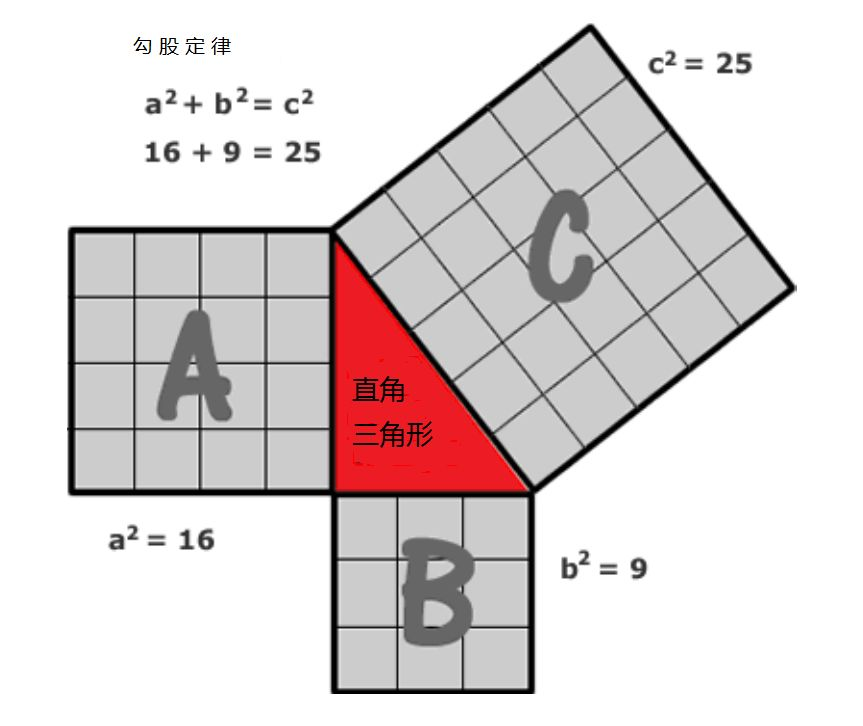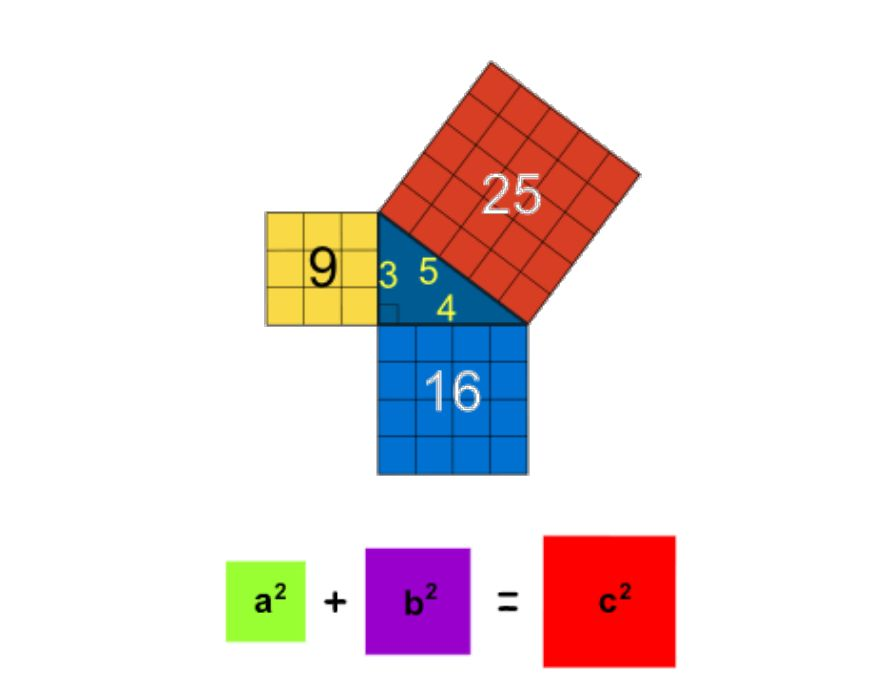v = s/t = sf = [1d- 空间 - 时间 ]

v = s/t = sf = [1d- 空间 - 时间 ] = [1d- 空间 ] = 线 / 矢量 ,

v&³2; = s&³2;/t&³2; = s&³2;f&³2; = [2d- 空间 - 时间 ] = [2d- 空间 ] = 面积 , t =1/f = 1

E = v&³2; = s&³2;/t&³2; = s&³2;f&³2; = [2d- 空间 - 时间 ]

E = SP(A)v&³2; = SP(A)s&³2;/t&³2; = SP(A)s&³2;f&³2; = SP(A)[2d- 空间 - 时间

E = EA f = SP(A)[1d- 空间 - 时间 ][1d- 空间 ] f,

E = mc&³2; = SP(A)[2d-space-time]p

E = SP(A)v&³2; = SP(A)s&³2;/t&³2; = SP(A)s&³2;f&³2; = SP(A)[2d- 空间 - 时间 ] = SP(A)[2d- 空间 ], t = 1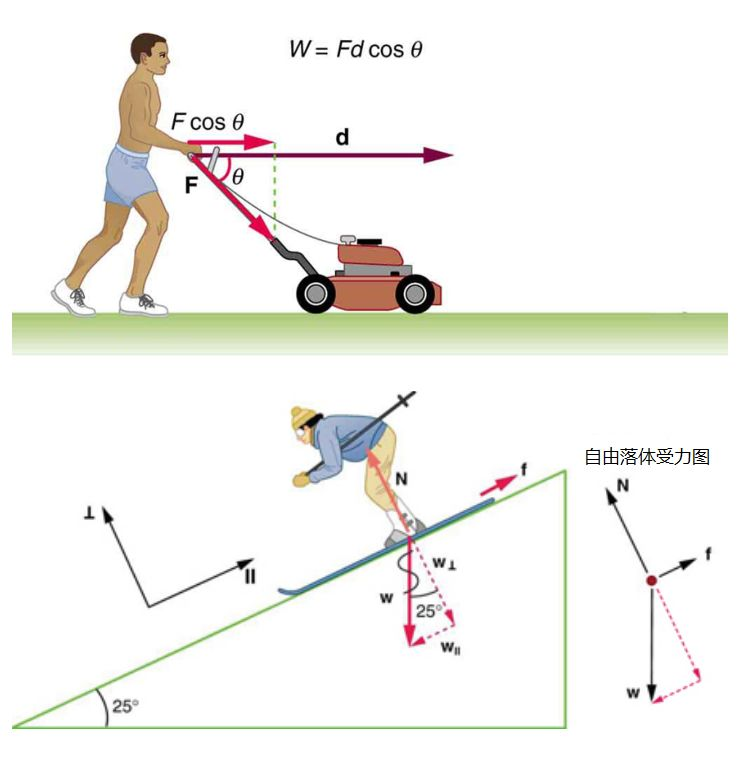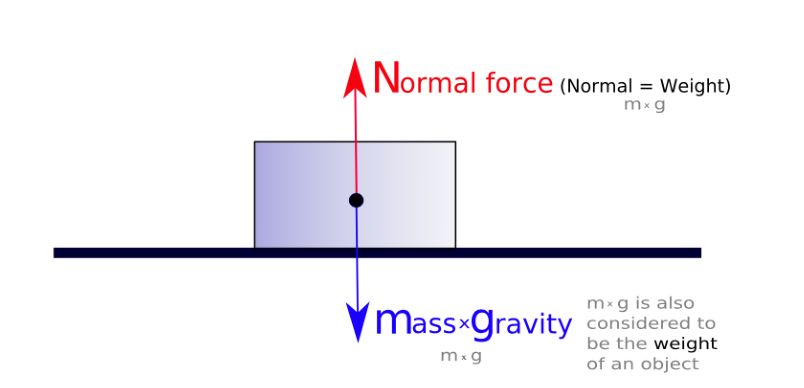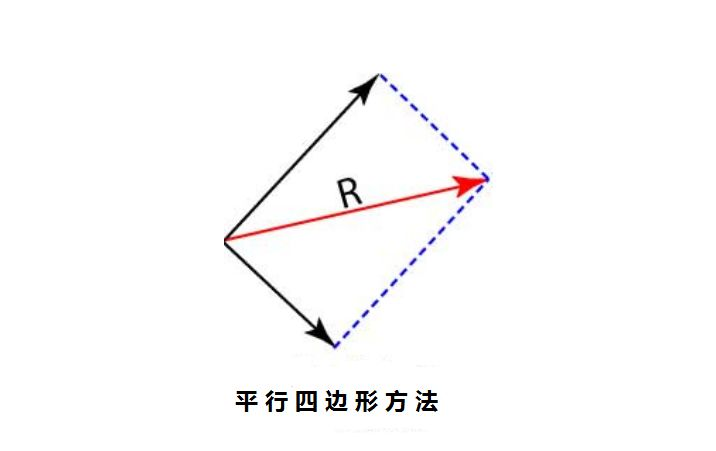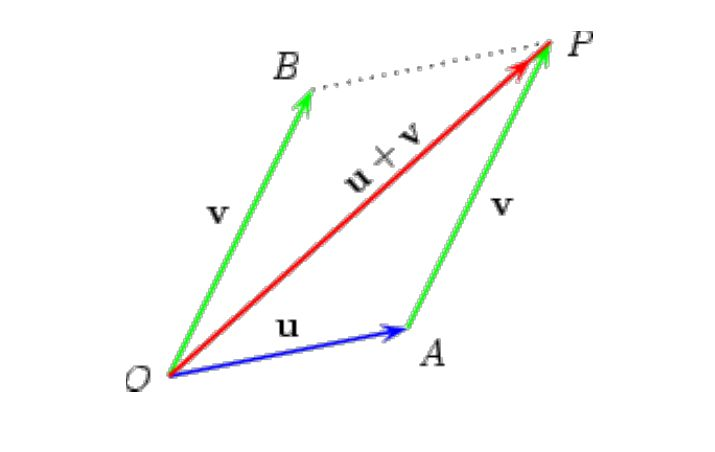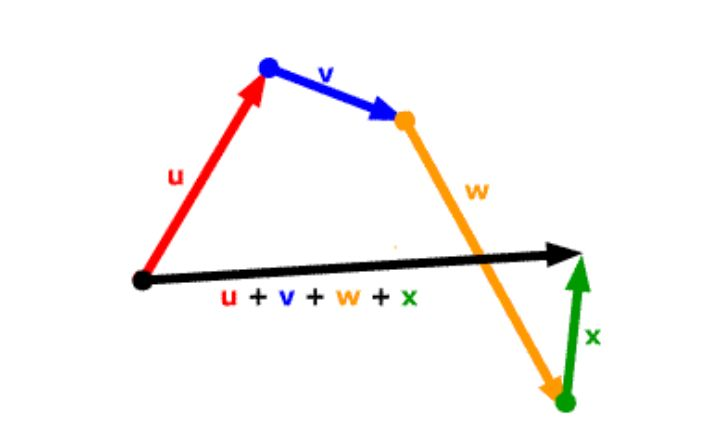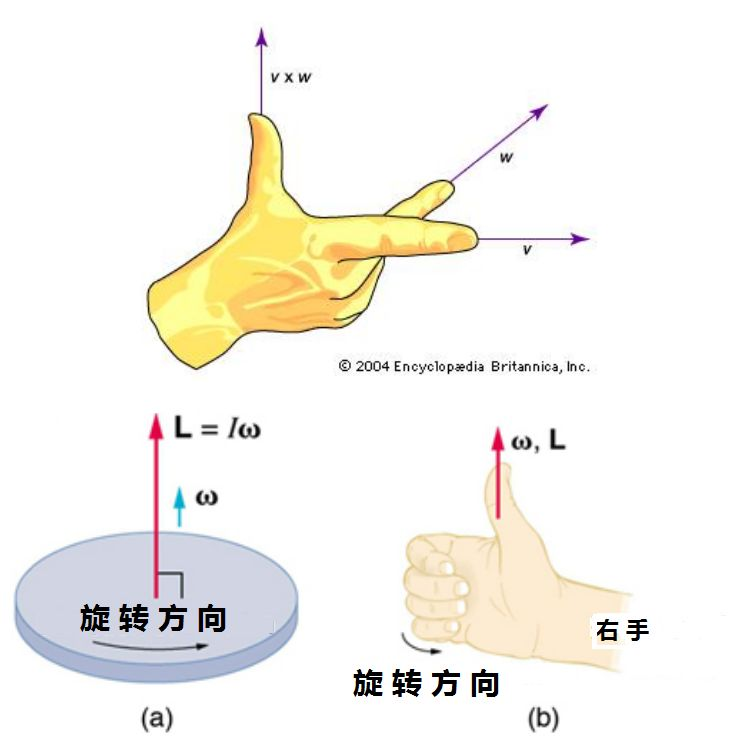未完待续

译自：斯坦科夫宇宙法则出版社

编译 | 马克兔文【相关阅读】